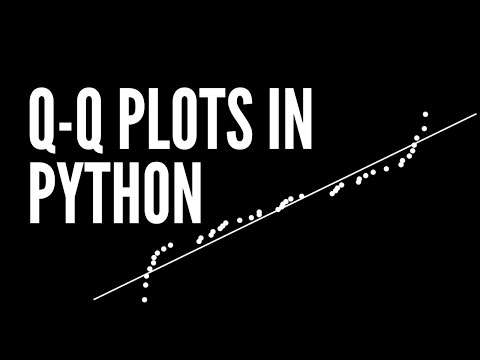# Quantile (Q-Q) Plots from Scratch In Pandas

In this tutorial, we will walk through a simple introduction to normal quantile-quantile (QQ) plots (a graphical method for assessing whether a set of observations is approximately normally distributed). I discuss the motivation for the plot, the construction of the plot, then look at several examples using python pandas.

## To view the video